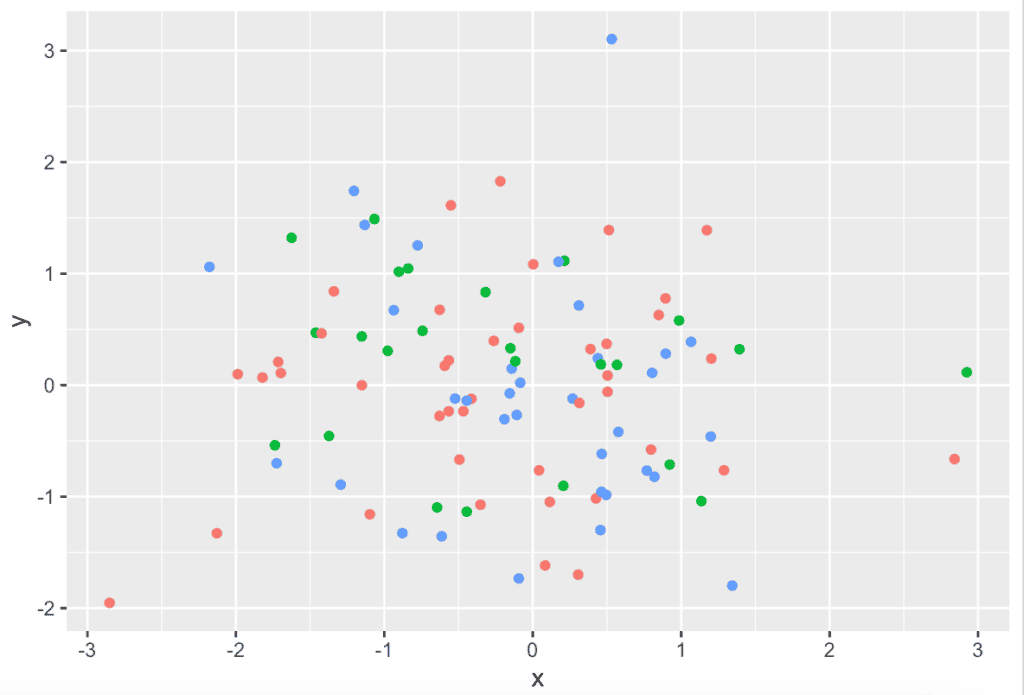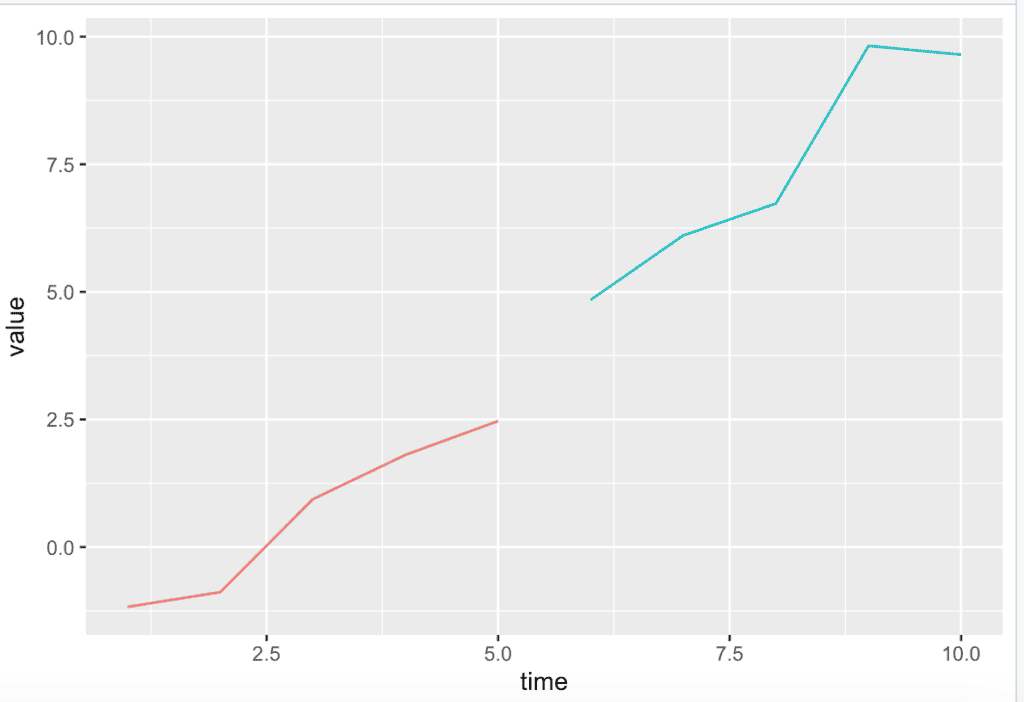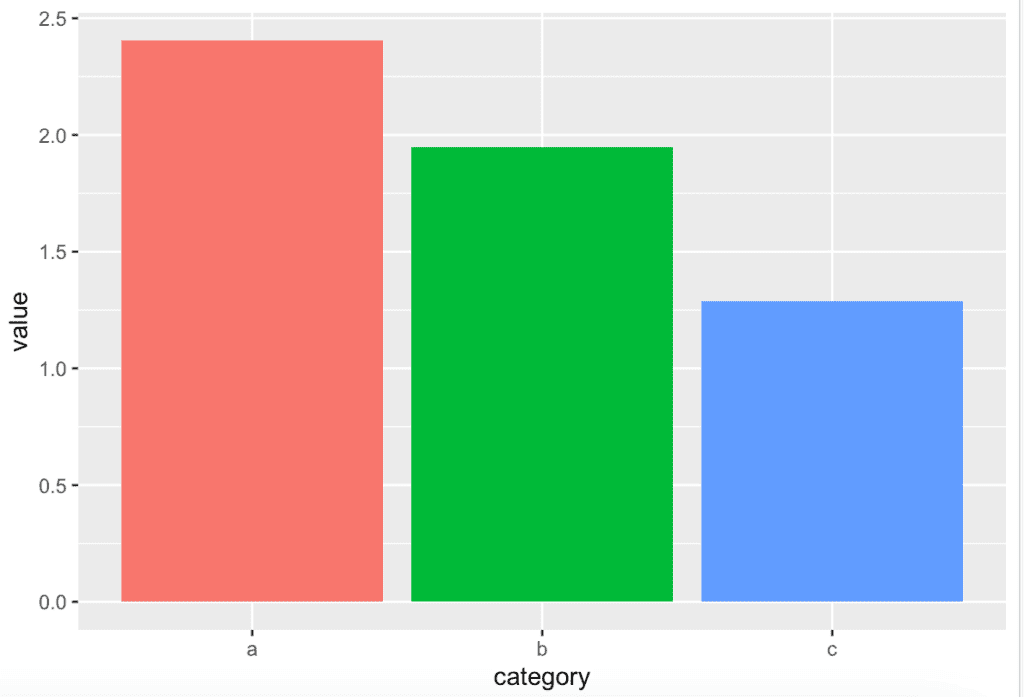# How to Remove a Legend in ggplot2 (With Examples)

To remove a legend from ggplot2 in R, use the “theme()” function along with the legend.position argument set to “none” to completely remove the legend from the plot.

## Example 1: Set legend.position = none

Here’s an example that demonstrates how to create a scatter plot without a legend:

``````library(ggplot2)

# Create a sample data frame
df <- data.frame(
x = rnorm(100),
y = rnorm(100),
category = sample(letters[1:3], 100, replace = TRUE)
)

# Create a scatter plot without a legend
plot <- ggplot(df, aes(x = x, y = y, color = category)) +
geom_point() +
theme(legend.position = "none")

# Display the plot
plot
``````

OutputBy adding theme(legend.position = “none”), we are instructing ggplot2 not to display the legend that would normally show the color mapping for the category variable.

## Example 2: Line Plot without Legend

``````# Load the necessary libraries
library(ggplot2)

# Create a sample data frame
df <- data.frame(
time = 1:10,
value = cumsum(rnorm(10)),
group = rep(1:2, each = 5)
)

# Create a line plot without a legend
plot <- ggplot(df, aes(x = time, y = value, color = as.factor(group))) +
geom_line() +
theme(legend.position = "none")

# Display the plot
plot``````

Output## Example 3: Bar Plot without Legend

``````# Load the necessary libraries
library(ggplot2)

# Create a sample data frame
df <- data.frame(
category = rep(letters[1:3], each = 4),
value = runif(12)
)

# Create a bar plot without a legend
plot <- ggplot(df, aes(x = category, y = value, fill = category)) +
geom_bar(stat = "identity") +
theme(legend.position = "none")

# Display the plot
plot``````

OutputUsing the fill aesthetic, we would typically have a legend showing the color mapping for the categories. However, we’re again using a theme(legend.position = “none”) to remove the legend from the plot.

That’s it!

## Related posts

How to Change the Legend Title in ggplot2

How to Change Legend Size in ggplot2

How to Change Legend Labels in ggplot2